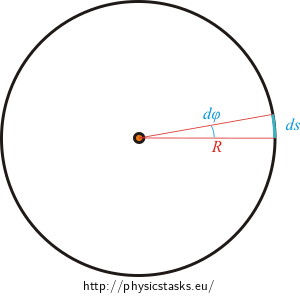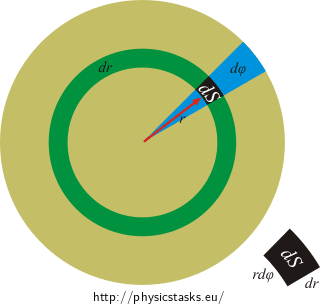## Moments of Inertia of a Ring and a Disc

Let us consider a thin disc and a thin ring.

A) First, try to guess without calculation, which shape, a disk or a ring, will have a greater moment of inertia if they have the same radius, mass and axis of rotation.

B) Determine the moment of inertia of a thin circular-shaped ring of mass m and radius R with respect to the axis passing perpendicularly through its centre.

C) Determine the moment of inertia of a thin circular disk of radius R and mass m with respect to the axis passing perpendicularly through its centre.

• #### Hint A

Think about the quantities on which moment of inertia of a body depends.

• #### Hint 1B

Review the theory explained in the task Moment setrvačnosti tyče. How can we use it in this task?

• #### Hint 2B

Think about the shape of the integral. Knowing the radius of the circle, how do we express the length of a small piece of the curve ds if we consider that it corresponds with an infinitely small angle ? Does this perspective make the determination of the limits of the integral easier?• #### Hint 1C

What is the difference in calculation of the moment of inertia of a disk and a ring? Will the results from the previous section be useful to us?

• #### Hint 2C

Express the moment of inertia of one ring. Use the results from the previous section.

Then we calculate the total moment of inertia of the disk by adding up the contributions of all the rings, i.e. by integration.

• #### Another possible solution of part C

The problem can also be very easily solved by the transformation into a double integral in polar coordinates:

$J_d = \int_{S} \sigma r^2 dS\,.$$dS = rd \varphi dr\,,$   $J_d = \int_{0}^{2\pi} \int_{0}^{R} \sigma r^3 dr d \varphi = \frac{1}{2}\pi \sigma R^4 = \frac{1}{2}m R^2\,.$
$J_r = mR^2\,.$
$J_d = \frac{1}{2} mR^2\,.$